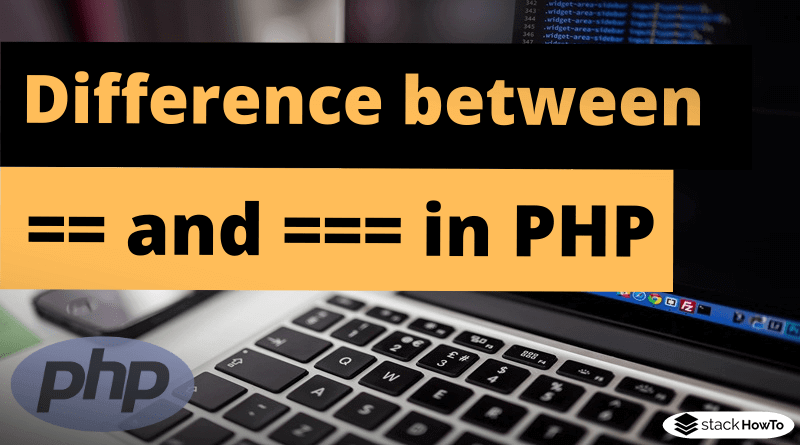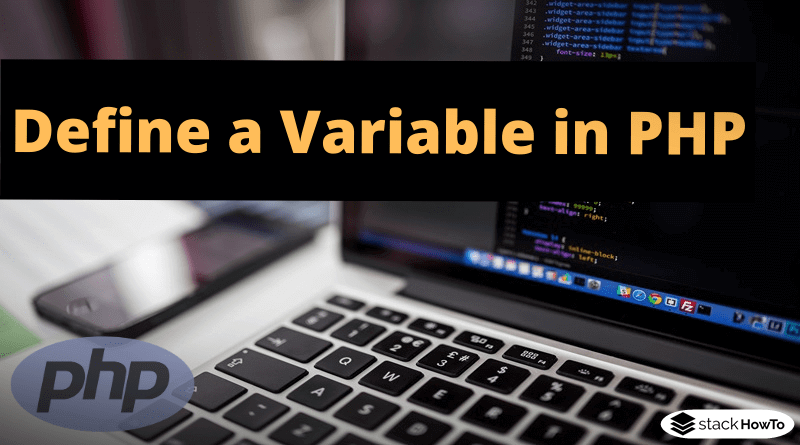MCQ

# PHP MCQ – Multiple Choice Questions and Answers – Basics – Part 2

This collection of PHP Multiple Choice Questions and Answers (MCQs): Quizzes & Practice Tests with Answer focuses on “PHP basic”.

###### 1. What is the result of the following PHP code?
```<?php
\$a = 1;
\$b = 2;
print \$a ."+". \$b;
?>```

A 1.+.2

B 1+2

C 3

D Error

B
.(dot) is used to concatenate two parts of the statement.

###### 2. Which is a newline character?

A /r

B /n

C \n

D \r

C
PHP deal with \n as a newline character.How to add a new line within echo in PHP?Line breaks are not automatically generated when outputting using echo(): <?php echo('A'); echo('B'); echo('C'); ?> Output: ABC Instead, it is necessary to set them manually.…Read More

###### 3. What is the result of the following PHP code?
```<?php
\$a = 1;
\$b = 2;
echo (\$a !== \$b);
?>```

A 1 !== 2

B Error

C 1

D False

C
!== operator returns 1 if \$a and \$b are different and \$a and \$b have the same type.What is the difference between == and === in PHPIn this tutorial, we’re going to see two ways to compare variables for equality in PHP.   The general behavior of “==” In most programming…Read More

###### 4. What is the result of the following PHP code?
```<?php
\$a  = "1";
\$b = "2";
print \$a + \$b;
?>```

A 12

B 3

C 1+2

D Error

B
The numbers within the double quotes are examined as integers and not string, so the value 3 is displayed and not 1+2.

###### 5. Which of the conditional statements below are supported by PHP?

A if statements

B if-else statements

C if-elseif statements

D switch statements

A, B, C, D
All statements are supported by PHP and all are used to determine different conditions during a program and take decisions on the basis of whether these conditions evaluate to true or false.

###### 6. Which of the following PHP declarations will store 5 in nbr?

A `5 = \$nbr;`

B `\$nbr = 5;`

C `int nbr = 5;`

D `int \$nbr = 5;`

B
You don’t need to indicate the datatype in PHP.How to Define a Variable in PHPVariables are “containers” that can store specified or determined values, similar to variables in mathematics. These values can be of different types, so-called data types.…Read More

###### 7. Which of the looping statements are approved by PHP?

A for loop

B while loop

C do-while loop

D foreach loop

A, B, C, D
All the loops above are supported in PHP as they can repeat the same block of code a given number of times, or until a particular condition is met.

###### 8. Which is the correct way of declaring a variable in PHP?

A \$2var

B \$_var

C \$this

D \$This

B, D
A variable in PHP can’t start with a number, also \$this is mostly used as reference to properties of a class so we can’t use \$this as a user define variable name.How to Define a Variable in PHPVariables are “containers” that can store specified or determined values, similar to variables in mathematics. These values can be of different types, so-called data types.…Read More

###### 9. What is the result of the following PHP code?
```<?php
\$name = "Thomas";
\$var = \$name;
echo "\$var";
?>```

A o

B m

C \$var

D Error

B
PHP deals with strings in the same way as arrays, allowing for specific characters to be accessed using array offset notation. In an array, index always starts from 0. So in the line `\$var = \$name;` if we count from start ‘m’ comes at index 3. So the output will be m.

###### 10. Which of the following statements is equivalent to `\$nbr += \$nbr`?

A `\$nbr = \$nbr`

B `\$nbr = \$nbr + \$nbr`

C `\$nbr = \$nbr + 1`

D `\$nbr = \$nbr + \$nbr + 1`

B
a += b is an addition assignment whose result is a = a + b. Identical to subtraction, multiplication, division, etc.MCQPractice competitive and technical Multiple Choice Questions and Answers (MCQs) with simple and logical explanations to prepare for tests and interviews.Read More